Random Art

Christopher A. Stone
Harvey Mudd College
stone@cs.hmc.edu
http://www.cs.hmc.edu/~stone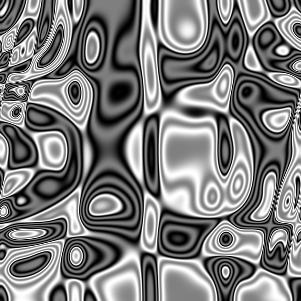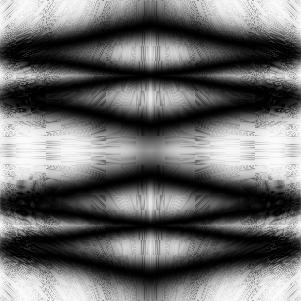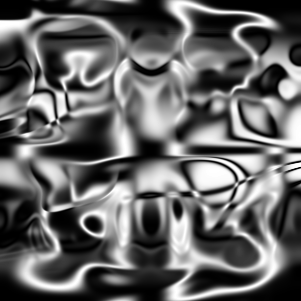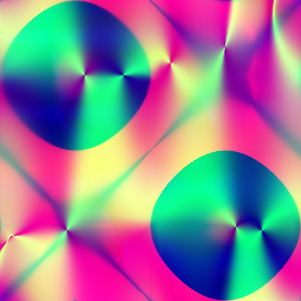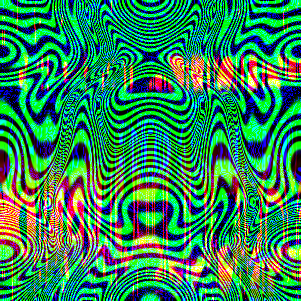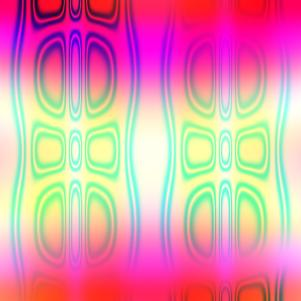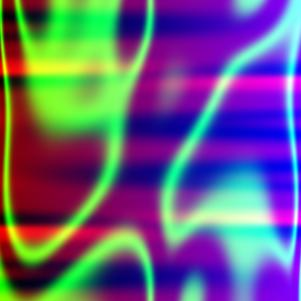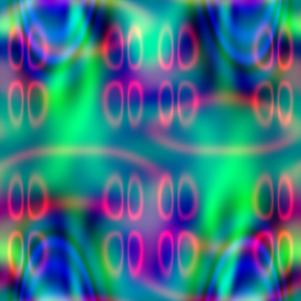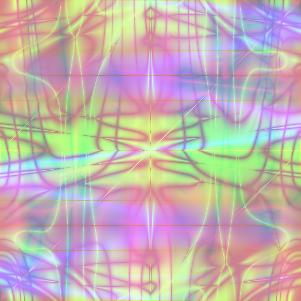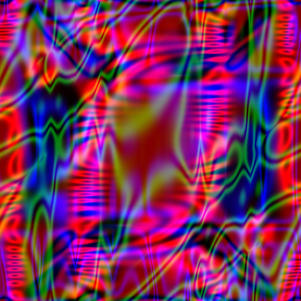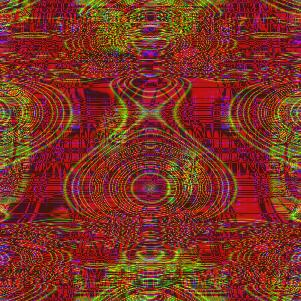Overview

Suppose we take an expression in x and y, built by nesting simple primitives: product, average, sin(pi*_), and cos(pi*_). These four operations map arguments in the range -1 to 1 to results in this same range. Thus, at any point with -1 <= x,y <= 1, our expression built from these primitives will return a value between -1 and 1. By scaling the answer to a grayscale value 0-255, we can plot the function in this 2-by-2 square. (From three such expressions, we can get red, green, and blue values for each point.)

Surprisingly, any sufficiently complicated expression has a decent chance of being interesting! We can generate deeply-nested expressions (8-10 levels of nesting seems to work well) completely randomly, see how they look, and keep the good ones.

This assignment was inspired by Andrej Bauer's Random Art.

 Summary Random Art Generator - large, randomly generated arithmetic expressions can yield surprisingly interesting pictures. Topics Representing, creating and using symbolic data (arithmetic expressions), with a bit of randomness. The particular variant used in our Programming Languages course adds in functional programming and higher-order functions, and alternate representations for symbolic data. Audience This has been used successfully in Programming Languages courses at several schools. However, because the core is just building and evaluating expressions (e.g., traversal of an abstract syntax tree), it should be easily adaptable for courses in the introductory sequence. Difficulty Easy to moderate Strengths The random-expression idea is simple but surprising. Students enjoy seeing the results; the best ones can be exhibited as a class gallery. There's great scope for variations and extensions, and not every part has a unique right answer. (For example, if you just generate the 6 expression forms is equally likely to be generated, then your whole expression will be just "x" or "y" 2/6 of the time; how should one generate expressions that are complicated enough, but not too complicated? Similarly, there's a lot of flexibility in choosing additional primitives.) This assignment has been used as a "second" exercise in functional programming (after students are very comfortable with writing reverse, append and other short functions). But it doesn't intrinsically require functional programming; a random art exercise could emphasize pointers and recursive tree traversals, the visitor or abstract factory patterns, seeds and pseudorandom number generation, or other topics. The assignment introduces the notion of symbolic data (a warmup to programs-as-data and to interpreters), without needing to bring in parsing. Weaknesses Because the outputs are random and unpredictable, some programming errors can't always be detected just by looking at generated pictures. (For example, the most common bug is leaving the "pi" out of the sine and cosine computations, which results in random but surprisingly boring pictures.) This can be mitigated by providing a fixed complicated expression whose correct picture is known. When asked to add new operators, many students default to raiding the language's built-in math library. This leads to lots of scaled-arctangents and square-roots-of-absolute-value. Being more explicit about the "creativity" requirement (e.g., requiring at least one ternary operation, and reminding them that operators need not be continuous) helps. The most difficult part for HMC students is representing expressions as functions. This could easily be omitted, depending on the purpose of the assignment. Dependencies A representation for expressions in x and y (trees, nested lists, closures, ...) is needed; evaluation of expressions usually requires some sort of tree traversal. Building expressions requires randomness. Output of pictures or picture files is also necessary, but this can be provided as skeleton code. The specific variant used in the HMC Programming Language course requires previous experience with Standard ML, including datatypes and signatures/structures; it also exercises first-class and higher-order functions. Variants Depending on how much supporting code is given, the assignment can be as simple as having students write code to build random expression trees and have them submit their favorite pictures. The students might also code the expression-evaluator. The HMC version supplies driver code to plot the function, but in a language with better built-in support for graphics than Standard ML, students could write the code to display the pictures. The assignment used in our programming language course brings in higher-order functions (implementing a simple for loop), and uses modularity and abstraction to allow two completely different representations of expressions (as trees and as first-class functions). Other representations are possible; in an untyped language like Python or Scheme, nested lists (e.g., ["Avg", ["SinPi", "X"], "Y"]) would be easy. The supplied procedure for generating color pictures could be improved. Random expressions yield a result in the range [-1,1]; mapping this into a 0-255 grayscale value is easy and yields pretty good results. The easiest way to get a color image is to generate three independent random expressions to compute R/G/B values at each point. Sometimes this works well, but frequently it looks like the superposition of three completely unrelated pictures. I occasionally urge highly-motivated students to consider how one might generate more coherent-looking results.

Materials

Programming Languages Assignment (Uses SML/NJ compiler and a converter for pbm/pgm files):

Python Variant (Uses PIL, the Python Imaging Library):

• Skeleton implementation: skel.py
• Precompiled implementation: art.pyo
"python art.pyo" generates 20 grayscale and 20 color pictures in .png format.

Other Examples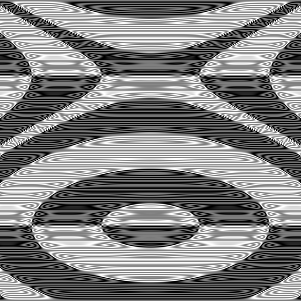sin(pi * sin(pi * sin(pi * (sin(pi * sin(pi * sin(pi * sin(pi * cos(pi * y))))) * cos(pi * sin(pi * cos(pi * avg(sin(pi * y), (x * x)))))))))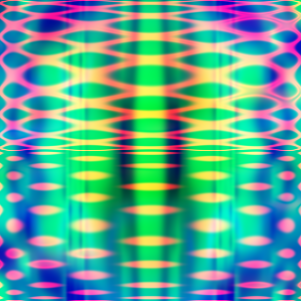red = sin(pi * avg((((cos(pi * (sin(pi * cos(pi * y)) * avg(avg(x, x), sin(pi * y)))) * avg(sin(pi * (sin(pi * y) * (y * x))), cos(pi * cos(pi * (y * y))))) * sin(pi * (sin(pi * (sin(pi * y) * sin(pi * y))) * cos(pi * ((y * y) * sin(pi * y)))))) * sin(pi * avg(cos(pi * avg(((y * x) * (x * x)), sin(pi * (y * x)))), sin(pi * avg(avg(sin(pi * x), avg(x, x)), sin(pi * avg(x, y))))))), cos(pi * cos(pi * avg(sin(pi * sin(pi * avg((x * x), (x * x)))), sin(pi * sin(pi * sin(pi * sin(pi * y)))))))))

green = sin(pi * ((avg(avg(cos(pi * (cos(pi * cos(pi * x)) * (cos(pi * x) * avg(y, x)))), ((cos(pi * cos(pi * y)) * (cos(pi * x) * (x * y))) * sin(pi * sin(pi * avg(y, y))))), cos(pi * (avg(sin(pi * sin(pi * x)), sin(pi * sin(pi * x))) * sin(pi * sin(pi * (x * y)))))) * avg((avg(cos(pi * sin(pi * cos(pi * x))), avg((sin(pi * x) * cos(pi * y)), avg(cos(pi * x), cos(pi * x)))) * avg(avg(sin(pi * cos(pi * x)), sin(pi * sin(pi * x))), (avg(cos(pi * x), avg(y, x)) * avg(sin(pi * y), sin(pi * x))))), (cos(pi * cos(pi * (avg(y, y) * (y * x)))) * cos(pi * cos(pi * sin(pi * avg(x, x))))))) * sin(pi * avg(avg(sin(pi * cos(pi * sin(pi * cos(pi * x)))), avg(sin(pi * cos(pi * cos(pi * y))), ((sin(pi * y) * (x * y)) * cos(pi * (y * y))))), cos(pi * avg(((cos(pi * y) * (y * y)) * avg(sin(pi * y), cos(pi * y))), (((x * x) * avg(y, x)) * cos(pi * sin(pi * x)))))))))

blue = avg(sin(pi * (avg(cos(pi * avg((cos(pi * (x * x)) * cos(pi * (x * y))), avg(avg((x * x), avg(y, y)), avg(cos(pi * y), cos(pi * x))))), avg(avg(avg((sin(pi * y) * (x * y)), sin(pi * (x * x))), avg(((x * x) * sin(pi * y)), (avg(x, x) * sin(pi * y)))), avg((cos(pi * sin(pi * y)) * cos(pi * avg(x, x))), sin(pi * avg(sin(pi * y), sin(pi * y)))))) * cos(pi * avg(avg(avg(sin(pi * (x * x)), avg(sin(pi * y), sin(pi * x))), cos(pi * avg(cos(pi * y), avg(y, x)))), (((avg(x, y) * cos(pi * x)) * cos(pi * avg(y, x))) * avg(cos(pi * (y * x)), ((x * x) * (y * x)))))))), avg(((((sin(pi * sin(pi * avg(x, x))) * avg(avg(sin(pi * y), sin(pi * y)), avg(avg(x, x), cos(pi * y)))) * sin(pi * sin(pi * sin(pi * (y * y))))) * avg(cos(pi * avg(avg(avg(x, y), (y * x)), cos(pi * sin(pi * x)))), (sin(pi * sin(pi * sin(pi * x))) * cos(pi * ((y * y) * cos(pi * x)))))) * avg(cos(pi * cos(pi * sin(pi * cos(pi * avg(x, y))))), (sin(pi * (cos(pi * avg(y, x)) * sin(pi * cos(pi * x)))) * ((sin(pi * cos(pi * y)) * avg(avg(x, x), cos(pi * x))) * avg((sin(pi * x) * avg(y, x)), sin(pi * sin(pi * x))))))), ((cos(pi * cos(pi * (sin(pi * (y * y)) * cos(pi * cos(pi * x))))) * avg(sin(pi * avg(cos(pi * sin(pi * y)), (cos(pi * x) * avg(x, x)))), cos(pi * cos(pi * cos(pi * avg(x, y)))))) * sin(pi * (avg((cos(pi * (y * y)) * cos(pi * sin(pi * y))), avg(((x * x) * sin(pi * x)), cos(pi * sin(pi * y)))) * avg(sin(pi * (avg(y, x) * avg(x, x))), cos(pi * avg((y * y), avg(y, y)))))))))